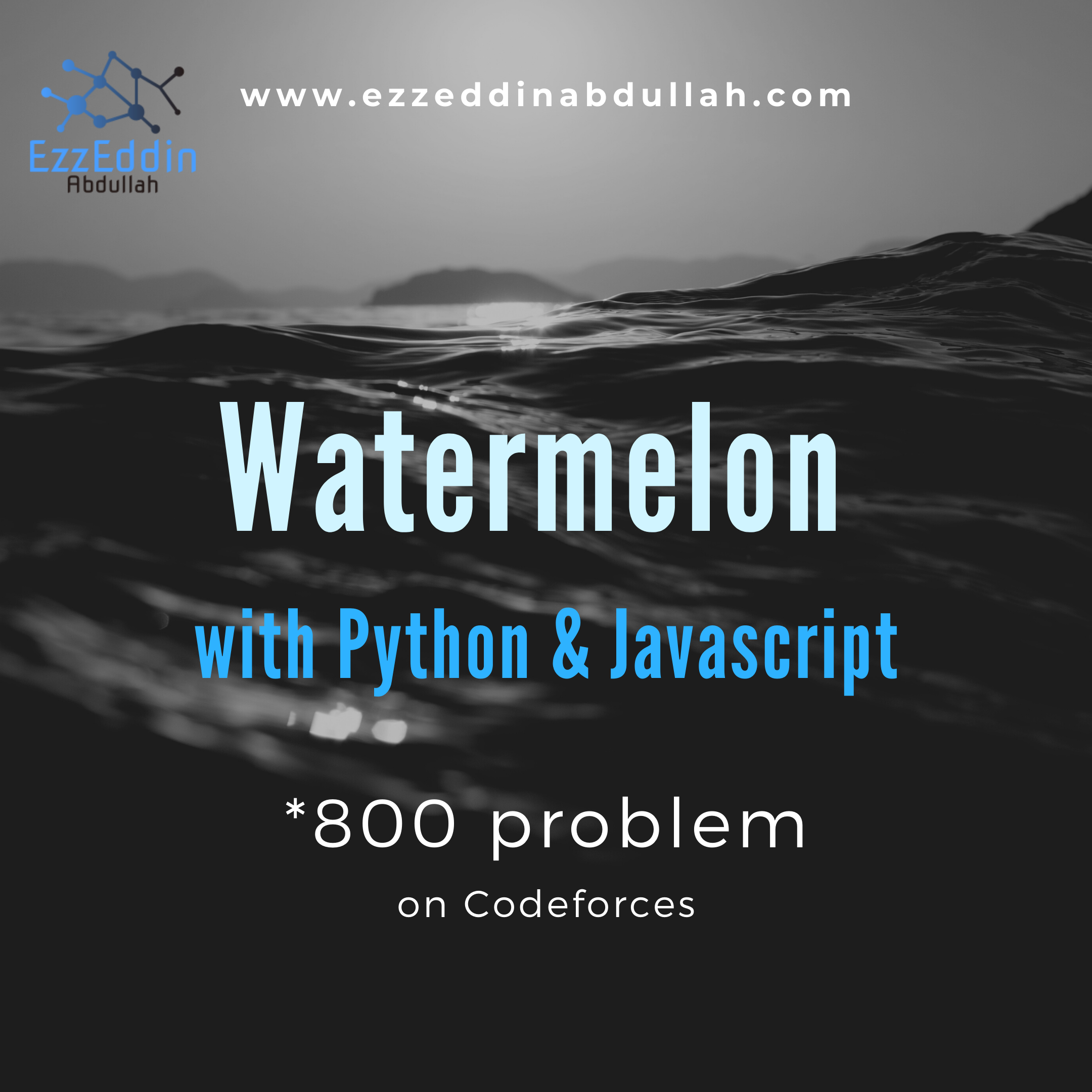# Watermelon with Python and Javascript

An *800 codeforces problem## Understanding the Problem

Watermelon is an *800 problem at codeforces which is the easiest problem out there. This problem basically states that there are Pete and his friend Billy who wants to the find the possibility to divide number of kilos in even numbers which means for example if there is 8 kilos, one way of dividing that number would be that Pete would take 2 kilos and Billy would take 6. Another possibility is 4 kilos for each of them.

## How to Think for a Solution

So this is a basic question of finding if the number of kilos is even in the first place; thus, you will find that it can be divisible to two even numbers.

But wait

There is a little exception, what if number of kilos is 2?

In that case, both Pete and Billy will have 1 kilo each.

So we have to exclude that from finding if number of kilos is even.